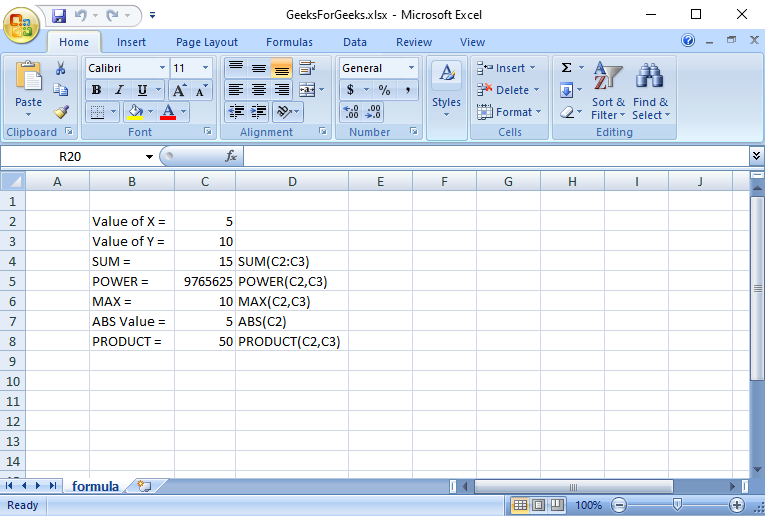Open in App
Not now

# How to Create a Formula in Excel using Java?

• Last Updated : 06 Dec, 2022

Apache POI is a popular open-source Java library that provides programmers with APIs for creating, modifying, and editing MS Office files. Excel is very excellent at calculating formulas. And perhaps most Excel documents have formulas embedded. Therefore, it’s trivial that on a fine day you have to deal with formulas when reading and writing Excel documents from Java. The good news is that the Apache POI library provides excellent support for working with formulas in Excel.

## Creating Formula in Excel using Java

We will use the Apache POI library in the project to write this article to share with you my experience in dealing with formulas in Excel files.

## Java

 `import` `java.io.FileNotFoundException;` `import` `java.io.FileOutputStream;` `import` `org.apache.poi.xssf.usermodel.XSSFCell;` `import` `org.apache.poi.xssf.usermodel.XSSFRow;` `import` `org.apache.poi.xssf.usermodel.XSSFSheet;` `import` `org.apache.poi.xssf.usermodel.XSSFWorkbook;`   `public` `class` `GFG {` `    ``public` `static` `void` `main(String[] args) ``throws` `Exception` `    ``{`   `        ``// Naming WorkBook` `        ``String excelfilename = ``"GeeksForGeeks.xlsx"``;`   `        ``// Creating Workbook` `        ``XSSFWorkbook workbook = ``new` `XSSFWorkbook();`   `        ``// Creating Spreadsheet` `        ``XSSFSheet spreadsheet` `            ``= workbook.createSheet(``"formula"``);`   `        ``// Create a row object using XSSFRow for creating` `        ``// Row` `        ``XSSFRow row;`   `        ``// Create a column object using XSSFCell for` `        ``// creating Cell` `        ``XSSFCell cell;`   `        ``// Creating row and start from 0 index value` `        ``row = spreadsheet.createRow(``1``);` `        ``// Creating column and start from 0 index value` `        ``cell = row.createCell(``1``);` `        ``// set Value of cell to apply formula` `        ``cell.setCellValue(``"Value of X = "``);` `        ``cell = row.createCell(``2``);` `        ``cell.setCellValue(``5.0``);`   `        ``row = spreadsheet.createRow(``2``);` `        ``cell = row.createCell(``1``);` `        ``cell.setCellValue(``"Value of Y = "``);` `        ``cell = row.createCell(``2``);` `        ``cell.setCellValue(``10``);`   `        ``row = spreadsheet.createRow(``3``);` `        ``cell = row.createCell(``1``);` `        ``cell.setCellValue(``"SUM = "``);` `        ``cell = row.createCell(``2``);`   `        ``// Creating SUM formula` `        ``cell.setCellFormula(``"SUM(C2:C3)"``);` `        ``cell = row.createCell(``3``);` `        ``cell.setCellValue(``"SUM(C2:C3)"``);`   `        ``row = spreadsheet.createRow(``4``);` `        ``cell = row.createCell(``1``);` `        ``cell.setCellValue(``"POWER ="``);` `        ``cell = row.createCell(``2``);`   `        ``// Create POWER formula` `        ``cell.setCellFormula(``"POWER(C2,C3)"``);` `        ``cell = row.createCell(``3``);` `        ``cell.setCellValue(``"POWER(C2,C3)"``);`   `        ``row = spreadsheet.createRow(``5``);` `        ``cell = row.createCell(``1``);` `        ``cell.setCellValue(``"MAX = "``);` `        ``cell = row.createCell(``2``);`   `        ``// Creating MAX formula` `        ``cell.setCellFormula(``"MAX(C2,C3)"``);` `        ``cell = row.createCell(``3``);` `        ``cell.setCellValue(``"MAX(C2,C3)"``);`   `        ``row = spreadsheet.createRow(``6``);` `        ``cell = row.createCell(``1``);` `        ``cell.setCellValue(``"ABS Value = "``);` `        ``cell = row.createCell(``2``);`   `        ``// Creating Absolute number formula` `        ``cell.setCellFormula(``"ABS(C2)"``);` `        ``cell = row.createCell(``3``);` `        ``cell.setCellValue(``"ABS(C2)"``);`   `        ``row = spreadsheet.createRow(``7``);` `        ``cell = row.createCell(``1``);` `        ``cell.setCellValue(``"PRODUCT = "``);` `        ``cell = row.createCell(``2``);`   `        ``// Creating SQRT formula` `        ``cell.setCellFormula(``"PRODUCT(C2,C3)"``);` `        ``cell = row.createCell(``3``);` `        ``cell.setCellValue(``"PRODUCT(C2,C3)"``);`   `        ``// This is a helpful wrapper around looping over` `        ``// allcells, and calling evaluateFormulaCell on each` `        ``// one.` `        ``workbook.getCreationHelper()` `            ``.createFormulaEvaluator()` `            ``.evaluateAll();`   `        ``// To make Auto size column` `        ``spreadsheet.autoSizeColumn(``1``);` `        ``spreadsheet.autoSizeColumn(``2``);` `        ``spreadsheet.autoSizeColumn(``3``);`   `        ``try` `{` `            ``// Place the output file in default location and` `            ``// also kept in try catch block` `            ``FileOutputStream outputfile` `                ``= ``new` `FileOutputStream(excelfilename);`   `            ``// Write to workbook` `            ``workbook.write(outputfile);`   `            ``// Close the output file` `            ``outputfile.close();`   `            ``// Display message for console window when` `            ``// program is successfully executed` `            ``System.out.println(excelfilename` `                               ``+ ``" is written successfully"``);` `        ``}` `        ``catch` `(FileNotFoundException e) {`   `            ``// Display error message for console window when` `            ``// program is not successfully executed` `            ``System.out.println(``"ERROR!! "` `+ e.getMessage());` `        ``}` `        ``finally` `{` `            ``workbook.close();` `        ``}` `    ``}` `}`

Output: On the console window

• When the program is successfully executed.

GeeksForGeeks.xlsx is written successfully

•  When the program is not successfully executed.

ERROR!! GeeksForGeeks.xlsx (The process cannot access the file because it is being used by another process)

Output: Workbook(excel file)My Personal Notes arrow_drop_up
Related Articles